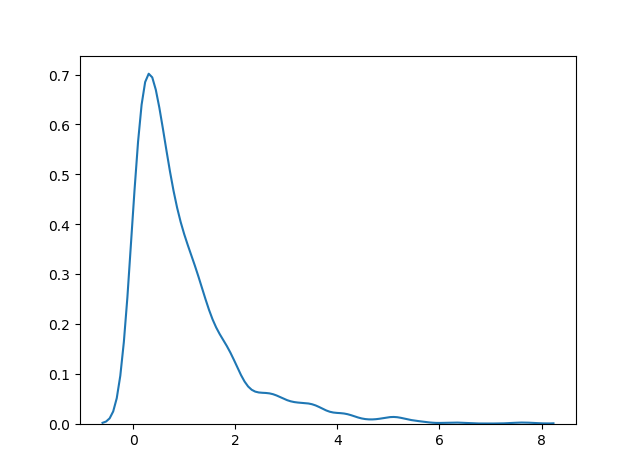THE WORLD'S LARGEST WEB DEVELOPER SITE

# Exponential Distribution

## Exponential Distribution

Exponential distribution is used for describing time till next event e.g. failure/success etc.

It has two parameters:

`scale` - inverse of rate ( see lam in poisson distribution ) defaults to 1.0.

`size` - The shape of the returned array.

### Example

Draw out a sample for exponential distribution with 2.0 scale with 2x3 size:

from numpy import random

x = random.exponential(scale=2, size=(2, 3))

print(x)
Try it Yourself »

## Visualization of Exponential Distribution

### Example

from numpy import random
import matplotlib.pyplot as plt
import seaborn as sns

sns.distplot(random.exponential(size=1000), hist=False)

plt.show()

### ResultTry it Yourself »

## Relation Between Poisson and Exponential Distribution

Poisson distribution deals with number of occurences of an event in a time period whereas exponential distribution deals with the time between these events.